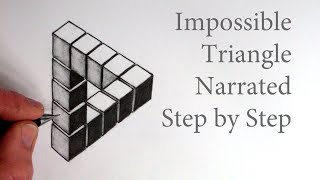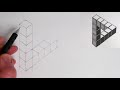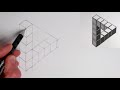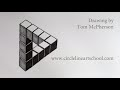# How to Draw The Impossible Triangle with 3d Cubes: Optical Illusion

210K+ views   |   5K+ likes   |   125 dislikes   |
Mar 03, 2018

### Thumbs### Transcription

• Hi, Tom here and in this week's Circle Line Art School video I will show you one way,
• a simple way, to draw the impossible triangle out of cubes,
• first draw a simple diamond shape, it needs to be wider than it is high and the diagonal
• lines need to be parallel to each other, they don't get wider
• or closer together, next draw a vertical line going down from its middle base and
• then we can draw two more parallel vertical lines, one on the left and one
• on the right, from the points on the left and right of the diamond, the original
• diamond that we drew, now we can divide this column of three lines into cubes,
• each cube is just made by drawing a diagonal line which is parallel to the
• diagonal line of the original diamond above it, we need in total five cubes, all
• stacked up on top of each other and each cube needs to be the same height as
• the one above it...
• next, from the baseline on the right, we can extend this baseline
• we can repeat this for the next two diagonal lines which go towards right
• extending them further off towards the right, now we can divide this shape into
• equal sized cubes with vertical lines and also diagonal lines that can link up
• with the diagonal lines on the left hand side of the original column of cubes
• that we drew, we need four extra cubes going in a row towards the right,
• next we can join the last of the two cubes on the right to the first cube
• that we drew, with three parallel diagonal lines
• from the second cube in
• our column we could extend the second and third diagonal lines to the right
• and this will create our last three cubes, next we can just erase two short
• lines and they need to be the right-hand side of the first cube, its base and the
• right hand side, the right hand vertical side, we can just tidy up the impossible
• triangle now a little bit too,
• now starting in the middle base of our
• diamond, we can darken this central line, next we can darken the left hand
• vertical line here, we can darken the left-hand baseline at
• the base, next to darken the two right-hand vertical cubes from our
• column, next we could darken the three diagonal lines on the right...
• and then the three diagonal lines from the diamond going right, we now have the
• impossible triangle a shape that you can draw in the two-dimensional surface of a
• piece of paper, but you can't really make it in 3d, it's an impossible shape in
• that sense, now we can make this impossible triangle into a series of
• cubes, to do this the first step is to make each of the lines which isn't an
• outside line of the triangle, into a double line, to separate all of the cubes
• that we already have, so they don't quite touch...
• as we do this we could also check and make sure that all of our diagonal lines
• are parallel to each other!
• in this drawing they're only actually three different types of lines sort of lines,
• there are vertical lines which will parallel, the diagonal lines to the left, which are all
• parallel to each other and then they're also diagonal lines to the right, which are
• also all parallel to each other...
• once you have doubled up all of the lines that separate the cubes, we can
• carefully erase the outside line just at the end of each of these double lines,
• then once we have done that we can add the missing bits in these little areas
• to each of the cubes to make it look 3d and separate from its neighbors, now we
• are ready to add some tonal values to this drawing, I think, with the impossible
• triangle you could see it as three "L" shapes so the first "L" shape could be a
• black tone, the second "L" shape could be a mid-tone and then the last sort of "L"
• shape could just be the white of the paper, thank you very much for watching
• this video, I hope you liked it and find it useful for your own drawings, if you'd
• www.circlelineartschool.com
• if you would like to keep up to date with all of the drawing tutorials on my youtube channel:
• Circle Line Art School, there are now over 270, please click the subscription button!
• Thank you very much for watching, keep drawing and see you next time!

### Description

Narrated step by step, Draw The Impossible Triangle out of Cubes. SUBSCRIBE: http://www.youtube.com/circlelineartschool New Drawing Tutorial every Saturday.
See how to draw the Impossible Triangle using 3D Cubes, narrated step by step.

Circle Line Art School: Episode #275 How to draw The Impossible Triangle with 3D Cubes

Thank you for watching this video, please subscribe to keep up to date with my weekly How to Draw art tutorials: https://www.youtube.com/circlelineartschool

Circle Line Art School

Hi, my name is Tom McPherson and I founded Circle Line Art School as an online art education resource for all. My aim is to inspire people to learn to draw and be more creative.

Thank you for your support and have a great day!

Tom McPherson

Circle Line Art School
https://www.circlelineartschool.com

How to Draw The Impossible Triangle using 3D Cubes Narrated Step by Step